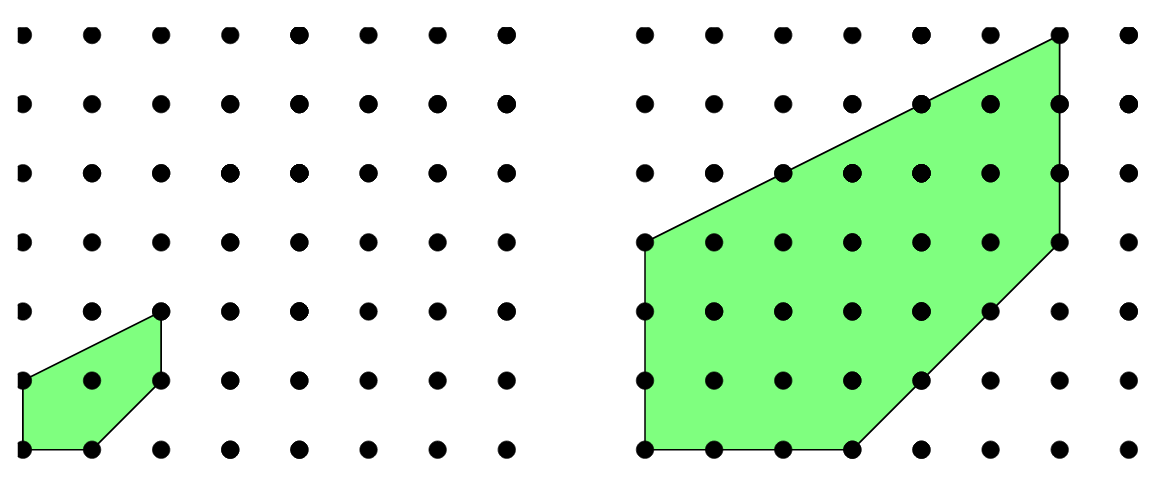# Counting solutions to equations

There are $$\binom{n-1}{m-1}$$ integer compositions of $$n$$ with $$m$$ parts. Complex polynomials of degree $$n$$ have $$n$$ zeros, counting multiplicity. Where else do we count solutions to equations? Our criteria are that the equations must be parametrized, and that for each parameter value there is a finite solution set. Some examples are given.

Integer solutions in a ball:

Theorem: If $$f$$ is a polynomial over $$\mathbb{Z}$$ in $$n$$ variables, let

$N(f, B) = |\{\mathbf{x} \in \mathbb{Z}^n: f(x_1, \ldots, x_n) = 0, \max_i |x_i| \leq B \}|.$

If $$f$$ is a singular homogeneous polynomial over $$\mathbb{Z}$$ of degree $$d$$ in $$n > (d-1)2^d$$ variables, then $$N(f, B) \sim c_f B^{n-d}, B \to \infty$$, under some technical conditions.

Diophantine equations:

Theorem: Say $$f$$ is a polynomial of degree $$d$$ over $$\mathbb{Z}_p$$ where $$GCD(p,d) = 1$$. If $$N(f)$$ is the number of solutions $$\mathbf{x} \in \mathbb{Z}_p^n$$ to $$f(\mathbf{x}) = 0$$, then $$N(f) = p^{n-1} + O(p^{n/2}), p \to \infty$$, assuming a non-singularity condition.

Non-negative integer solutions to linear equations:

E.g. $$\{ (x,y,z) : 3x + 5y + 17z \leq \lambda, x \geq 0, y \geq 0, z \geq 0 \}$$.

Theorem: Let $$\Delta(\lambda) = \{ \mathbf{x} \in \mathbb{Z}^n: M \mathbf{x} \leq \lambda\mathbf{b} \}$$. Then $$|\Delta(\lambda)|$$ is a polynomial in $$\lambda$$ of degree $$n$$.

Note that wlog $$\mathbf{b}$$ takes possible values $$-1,0,1$$. If not, multiply $$b_i$$ and $$[M]_{i,*}$$ by $$\textrm{lcm}(\mathbf{b})/b_i$$ and set $$\lambda' = \lambda / \textrm{lcm}(\mathbf{b})$$.

If $$\mathbf{b}$$ takes possible values $$-1,0,1$$, we may take the difference $$\Delta(\lambda) \setminus \Delta(\lambda -1)$$ to get solutions to an equality.(Above: Example solution sets for different values of $$\lambda$$.)

Locally restricted words over finite groups:

Theorem: If $$G$$ is a finite group and $$x_1, \ldots, x_m \in G$$, let $$N(m, a)$$ be the number of solutions to $$x_1 \cdots x_m = a$$ such that $$(x_1, \ldots, x_m)$$ satisfies a local restriction. Then under some conditions, as $$m \to \infty$$ we have $$N(m, a) \sim N(m, e)$$, where $$e \in G$$ is the identity element.

* * *